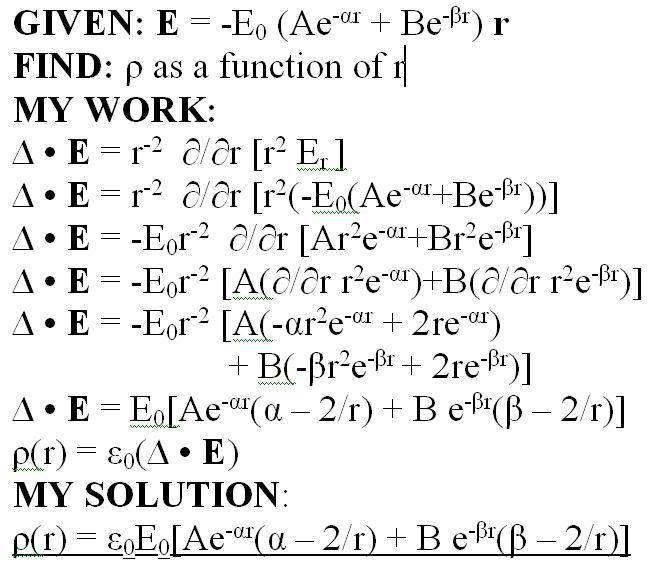# Electrostatics charge density

## Homework Statement

I didn't know how to display a lot of the symbols, so I wrote the problem out in microsoft word, took a screenshot, uploaded it to photobucket, and linked it.
Basically, I have to find the charge density as a function of r given the electrostatic field in the image. Any constants are positive. Bold means it's a vector; ignore the green underline; the del function is upside down - sorry about that.http://img.photobucket.com/albums/v696/talimtails/aphysicswork.jpg

My questions are..
1: Is my work method correct? i.e. is this how you solve for charge density?
2. Is there a way to simplify it more?
3. Is E0 a constant or not? The problem doesn't really say and is ambiguous to me. If it's not, how should I approach it? Thanks.
In all cases... please don't tell me if my answer is right or wrong. YES/NO answers are very much appreciated.

## Homework Equations

Relevant equations I used in the image

## The Attempt at a Solution

My attempt is in the image. ^_^

Last edited:

## Answers and Replies

First, is the vector \vec{r} in E meant to be the *unit* vector in the r-direction, or not?

Last edited:
Not the gradient, but the divergence. You cannot take the gradient of a vector field.

1. The approach is correct.

2. I don't think your final answer can be simplified, but I haven't checked your math.

3. I think you can assume that E0 is constant, no reason to expect it not to be. If it is not, you have to know how it varies with r, theta and phi.

Yes, r at the end in bold is the radial unit vector
I took the divergence of E and assumed spherical for the vector derivative

Thanks for your help!